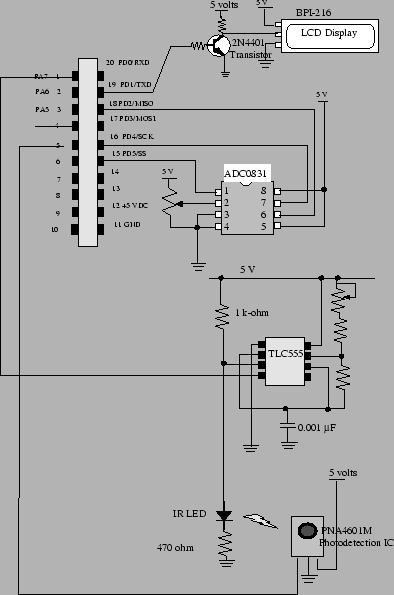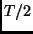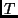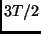Next: What you should have Up: Input Capture Interrupts Previous: Simplified Serial Communication Protocol

## Learning Module Exercise

In this learning module you'll build a system that uses an ADC chip to measure the voltage over a potentiometer. The ADC chip you'll use is the ADC0831. The quantized voltage will be transmitted to the MicroStamp11 over an SPI serial link. Your system will then transmit this quantized voltage over a wireless IR link and your MicroStamp11 will decode the received frame of data. The value transmitted and received by your MicroStamp11 will be displayed on a serial LCD display (BPI-216). Note that the ADC and LCD subsystems were already built in the earlier learning module covering serial communication.

Figure 54 shows the hardware schematic diagram for your system. This schematic was obtained by composing the individual schematic diagrams we showed earlier for the ADC, LCD, and wireless IR link subsystems.The software side of this project is shown in the following listing.

 #include "kernel.c"
void main(void){
int i;
init();

OutChar(254);OutChar(1);

while(1){
sdata = shiftin(SPI_62kHz);
sdata &= ~0x80;

OutChar(254);OutChar(2);
OutString("S=");OutUDec(sdata);
OutChar(SP);OutChar(SP);OutChar(SP);
for(i=0; i<8; i++){
if((sdata & bit(i))==0){
OutChar('0');
}else{
OutChar('1');
}}
OutChar(SP);OutChar(SP);OutChar(SP);

if(_sbit == 0){
set_pin(1);
asm(" sei");
TMSK1 |= OC4;
TOC4=TCNT + _bit_time;
TFLG1 |= OC4;
asm(" cli");
}

OutChar(254); OutChar(192);
OutString("R=");OutUDec(rdata);
OutChar(SP);OutChar(SP);OutChar(SP);
for(i=0;i<8;i++){
if((rdata & bit(i))==0){
OutChar('0');
}else{
OutChar('1');
}}
OutChar(SP);OutChar(SP);OutChar(SP);
}
}
#include"vector.c"


The preceding program consists of a while loop that has four parts. The fist part using the shiftin instruction shifts in the converted voltage from the ADC chip into the variable sdata. The variable sdata contains the byte that is to be transmitted over our wireless link. The next part of the main program displays the transmitted data in sdata on the LCD display. The LCD display shows both the decimal and binary versions of sdata. The third part of the program arms the OC4 interrupt. In this program we'll use the OC4 interrupt to automatically configure and transmit the serial frame containing sdata. The actual code controlling the transmission of data will be found in the OC4 interrupt handler. The final part of the program displays the received data on the LCD display. The received data is held in the global variable rdata. Our program displays both the binary and decimal versions of rdata. Note that the main program doesn't contain the code that actually decodes the received serial frame. The code that fills the variable rdata will be found in the interrupt handlers for the IC1 and OC3 event. In this program, we coordinate the interaction of these two hardware events in order to extract rdata from a received frame of data.

The majority of the work in this learning module is performed by the interrupt handlers. So we now turn to the interrupt handlers found in kernel.c. We first look at the OC4 interrupt handler. Recall that we arm this handler immediately after a converted voltage has been shifted into the MicroStamp11. The code segment for the handler is shown below:

 unsigned int _sbit;
unsigned int sdata;
unsigned int _bit_time, _bit_time_2;

#pragma interrupt_handler OC4han()
void OC4han(void){
if(_sbit==0){
TOC4 = TOC4 + _bit_time_2;
PORTA &= ~bit(7);
}
if(_sbit==1){
TOC4=TOC4 + _bit_time_2;
PORTA |= bit(7);
}
if((_sbit > 1) & (_sbit<10)){
TOC4=TOC4+_bit_time;
if( (sdata & bit(_sbit-2))==0){
PORTA |= bit(7);
}else{
PORTA &= ~bit(7);
}
}
if(_sbit==10){
TOC4 = TOC4 + _bit_time;
PORTA &= ~bit(7);
}
if(_sbit==11){
_sbit=0;
TMSK1 &= ~OC4;
PORTA &= ~bit(7);
}else{
_sbit=_sbit+1;
TFLG1 |= OC4;
}
}

extern void OC4han();
void (* OC4_handler[])()= { OC4han };


The OC4 interrupt handler uses the global variable _sbit to control its operation. This variable is used to record which part of the frame is currently being transmitted. If _sbit equals zero, then we know that we are starting the frame. So we set the output high for a period ofseconds. The actual durationis set in the variable _bit_time. The durationis stored in the variable _bit_time_2. This first part transmits the start-bit. After the start bit is transmitted, we set the line low forseconds. This completes the transmission of the start bit. The handler then begins sending out the information bits. After the last information bit is sent, our handler transmits a stop bit of durationseconds. When the stop bit transmission is complete, then the OC4 handler disarms itself. It won't be re-armed until the next AD conversion takes place.

The OC4han ISR automatically transmits the converted byte in sdata over the wireless link. The reception and decoding of this frame of data is done by the coordinated use of an input capture and output compare interrupt. The input capture interrupt is used to detect the start-bit. Once the start bit has been detected, then the IC interrupt handler arms the OC3 interrupt event. The OC3 interrupt is used to read off 8 information bits that are assumed to be separated byseconds. The source code for the IC1 interrupt handler is shown below.

 unsigned int _rbit;
unsigned int rdata, rdata_buf;
unsigned int _start_frame;
unsigned int _start_time;
unsigned int _start_bit_width;

#pragma interrupt_handler IC1han()
void  IC1han(void){
asm(" sei");
if(TCTL2 == 0x10){
TCTL2 = 0x20;
_start_frame = TIC1;
TFLG1 |= IC1;
}else{
if(_start_frame < TIC1){
_start_bit_width = TIC1 - _start_frame;
}else{
_start_bit_width = 65536 - _start_frame + TIC1;
}
if(_start_bit_width < (_bit_time_2 + 1000)){
TOC3 = _start_frame + _start_time;
rdata_buf = 0xFF;
TMSK1 &= ~IC1;
TMSK1 |= OC3;
TFLG1 |= OC3;
}else{
TCTL2 = 0x10;
TFLG1 |= IC1;
}
}
asm(" cli");
}

extern void IC1han();
void (* IC1_handler[])()={ IC1han };


The IC1 handler is first called on the rising edge of a received pulse on pin PA2. At this time, we store the current time in _start_frame and set the next interrupt to occur on the falling edge. So the next time the IC1 ISR is called, we know that it was triggered by the falling edge of the pulse. We then compute the width of this pulse and store it in _start_bit_width. If the size of _start_bit_width is consistent with a start bit of durationseconds, then we disarm the IC1 interrupt and enable the OC3 interrupt. For the OC3 interrupt, we set its control register TOC3 so it triggersseconds after the rising edge of the start bit. If the received pulse width is not consistent with the width of a start bit, we then reset the IC1 interrupt so it begins looking for a rising edge again.

The IC1 interrupt is used to detect the start bit. After the start bit is detected, the function IC1han starts an output compare timer (OC3). The output compare interrupt OC3 is used to read off the information bits in the received frame. The source code for the OC3 interrupt handler is shown below.

 #pragma interrupt_handler OC3han()
void OC3han(void){

if(_rbit < 8){
if((PORTA & bit(2))==0){
rdata_buf &= ~bit(_rbit);
}else{
rdata_buf |= bit(_rbit);
}
_rbit = _rbit+1;
TOC3 = TOC3 + _bit_time;
TFLG1 |= OC3;
}else{
_rbit=0;
TMSK1 &= ~OC3;
TMSK1 |= IC1;
TFLG1 |= IC1;
TCTL2 = 0x10;
rdata= rdata_buf;
}
}

extern void OC3han();

The OC3 interrupt handler simply reads the input pin PA2 and sets the appropriate bit in rdata. (Actually we construct rdata in a temporary buffer rdata_buf. When the frame is completely decoded, then we move rdata_buf into rdata.) The handler then resets the control register TOC3 so that the next information bit is readseconds later. After the OC3 interrupt has been called 8 times, then we know that all of the information bits have been read. At this point, we can then reset _rbit, disarm the OC3 interrupt and re-arm the IC1 interrupt so we are ready to receive the next start bit.